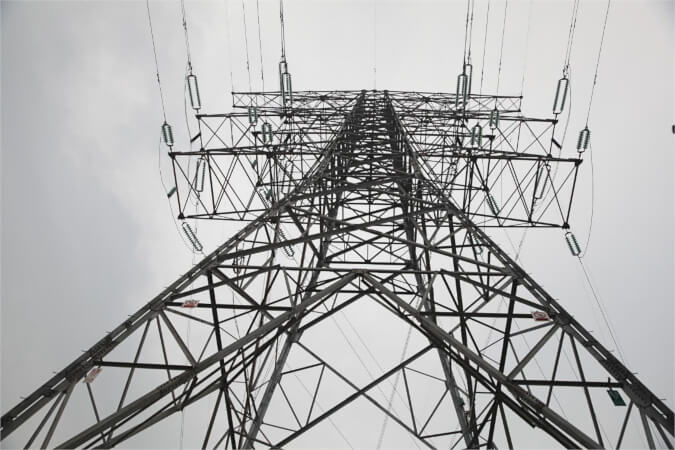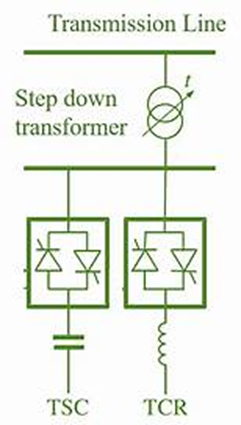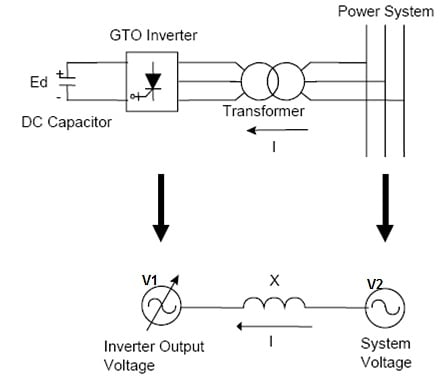Technical Article

# Introduction to STATCOM Systems

July 31, 2023 by Kevin Clemens

## Modern power electronics provide keys to improving the performance and reliability of electrical power grids.

Flexible alternating current transmission systems (FACTS) use solid-state power electronics to enhance control and improve the power transfer performance of electrical power grids. Transmission inefficiencies account for between 8 and 9 percent of energy loss in a grid, and using FACTS to regulate voltage, improve power factors, regulate harmonics, and stabilize the entire system results in a better and more efficiently run grid system.### AC Power Flow

A static synchronous compensator (STATCOM) uses modern power electronics to control power flow through alternating current (AC) transmission networks, improving the stability and reliability of electrical power grids. Sometimes known as a static synchronous condenser (STATCON), the device acts as a shunt—it creates a low-resistance path for electric current to pass through the circuit. These devices fit in the category of static VAR compensators (SVC) that use modern power electronics to regulate grid voltage.

Electrical circuits power two types of electrical loads—reactive and non-reactive (or resistance) loads. Pure resistance loads (an electrical heater, for example) do not store electric energy and result in no phase shift between voltage and current when applied to an AC power system. Reactive loads such as transformers, motors, or electrical circuits containing inductors or capacitors store electrical energy through magnetic fields and electrostatic charges and exhibit a reactive power flow depending on the phase shift between voltage and current in AC systems.

The power in an electrical system (P) equals the voltage V times the current I (or P = VI with P measured in watts.) In AC networks, a phase difference (δ) between the voltage and the current can exist, which causes a power factor of less than 1. The out-of-phase power is called reactive power and is designated volt-amp reactive (VAR)—the unit adopted in 1930 for reactive power.

In an AC system, the power factor is defined by the ratio of real power used by a load to the apparent power in the circuit—A system with a lower power factor will have higher amounts of energy storage in the load, reducing overall transmission efficiency.

### Static VAR Compensators

STATCOMs fall into a larger class of devices, called static VAR compensators when used as part of a FACTS power grid. SVCs are attached to the transmission grid to regulate the voltage through capacitors or inductors. The key to the creation of STATCOM devices was the development in the 1990s of gate turn-off thyristors (GTO) by General Electric.

An AC electrical power grid consists of combinations of resistance and reactive loads and the grid's voltage can be regulated using reactive power. Because the load on the grid at the consumer end can vary from second-to-second regulation devices are needed to detect these changes and control the amount of reactive voltage in the system.

Let’s examine how reactive power regulates the voltage in a power grid. Recall the power in an electrical system P is equal to the voltage V times the current I, or P = VI, so if the load at the consumer end increases, it draws more current (I) from the grid, causing the voltage (V) to drop with a constant power (P) level. To operate properly, the load also needs its rated voltage. That’s where the reactive voltage comes into play. A regulation device (like a STATCOM) detects the impending voltage drop, switching in capacitor banks and increasing the reactive voltage in the system to raise the voltage at the load to the rated value. Likewise, a decrease in the load at the consumer end results in a reduction of the reactive voltage component, and inductors are switched in, lowering the system voltage at the load to its rated level.### Adding or Absorbing Reactive Power

The thyristor-controlled STATCOM device continuously adds or absorbs reactive power to maintain the grid voltage stability. The phase angle δ between the voltage V1 of the STATCOM and V2 of the grid determines the magnitude of the contribution of voltage support to the grid.##### V1 is the voltage provided by the STATCOM device, while V2 is the voltage of the transmission grid. Image used courtesy of Electrical Concepts

In the above figure, the controlling equation for reactive power flow Q is:

$Q=\Big(\frac{V2}{X}\Big)[V1cos\delta-V2]$

If the phase angle δ=0, then the flow of active power becomes zero and the flow of reactive power depends on the voltage difference between the STATCOM and the grid (V1 – V2). This provides two possibilities:

1.) If V1 is greater than V2, reactive power flows from the SATCOM V1 to the grid V2

2.) If V2 is greater than V1, reactive power will flow from the grid V2 to the STATCOM device V1

STATCOM voltage source converters (VSCs) using square-wave inverters with GTO thyristors control the output AC voltage by changing the DC capacitor input voltage—this makes the converter output voltage proportional to the DC voltage. A transformer couples the output of the VSC to the grid, neutralizing harmonics contained in the square waves produced by the inverter. More recently, other high-power semiconductor devices, such as insulated gate bipolar transistors (IGBT) and integrated gate commutation thyristors (IGCT), have found applications in STATCOM devices.

Because the voltage source of a STATCOM is created from a DC capacitor, the device has limited active power capability. This can be increased, however, if a larger-scale energy storage device is connected across the DC capacitor, allowing the STATCOM to independently send out or absorb reactive power, further improving grid controllability.

### Distributed Energy Resources

Electrical power grids look different today than in past decades as distributed energy resources like intermittent wind and solar and ever-increasing battery energy storage systems add variability to traditional utility services. Maintaining safe, stable, and reliable grids has required the development of tools to enable the analyses of networks and the creation of automatic systems to provide near-instantaneous control of grid parameters. The continuing development of power-electronics-based shunt reactive power compensation devices, like STATCOMS, will continue to improve the performance and reliability of the nation’s electrical power grid.